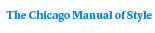# Navier-Stokes Equations200 pages | 5-1/4 x 8 | © 1988
Paper \$32.00 ISBN: 9780226115498 Published July 1989
E-book \$32.00 About E-books ISBN: 9780226764320 Published April 2020
Both an original contribution and a lucid introduction to mathematical aspects of fluid mechanics, Navier-Stokes Equations provides a compact and self-contained course on these classical, nonlinear, partial differential equations, which are used to describe and analyze fluid dynamics and the flow of gases.
Table of Contents• Contents
Contents
Introduction
1. Notation and Preliminary Material
2. The Stokes equations. Existence and Uniqueness of Weak Solutions
3. Regularity of Solutions of the Stokes Equations
4. The Stokes Operator
5. The Navier-Stokes Equations
6. Inequalities for the Nonlinear Term
7. Stationary solutions to the Navier-Stokes Equations
8. Weak Solutions of the Navier-Stokes Equation
9. Strong Solutions
10. Further Results Concerning Weak and Strong Solutions
11. Vanishing Viscosity Limits
12. Analyticity and Backward Uniqueness
13. Exponential Decay of Volume Elements
14. Global Lyapunov Exponents. Hausdorff and Fractal Dimension of the Universal Attractor
15. Inertial Manifolds
Bibliography
Index